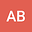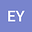Non-linear Least Square Fitting Technique for the Determination of Field Line Resonance Frequency in Ground Magnetometer Data: Application to Remote Sensing of Plasmaspheric Mass Density
•••• +1## Abstract

The accurate determination of the Field Line Resonance (FLR) frequency of a resonating geomagnetic field line is necessary to remotely monitor the plasmaspheric mass density during geomagnetic storms and quiet times alike. Under certain assumptions the plasmaspheric mass density at the equator is inversely proportional to the square of the FLR frequency. The most common techniques to determine the FLR frequency from ground magnetometer measurements are the amplitude ratio and phase difference techniques, both based on geomagnetic field observations at two latitudinally separated ground stations along the same magnetic meridian. Previously developed automated techniques have used statistical methods to pinpoint the FLR frequency using the amplitude ratio and phase difference calculations. We now introduce a physics-based automated technique, using non-linear least square fitting of the ground magnetometer data to the analytical resonant wave equations, that reproduces the wave characteristics on the ground, and from those determine the FLR frequency. One of the advantages of the new technique is the estimation of physics-based errors of the FLR frequency, and as a result of the equatorial plasmaspheric mass density. We present analytical results of the new technique, and test it using data from the Inner-Magnetospheric Array for Geospace Science (iMAGS) ground magnetometer chain along the coast of Chile and the east coast of the United States. We compare the results with the results of previously published statistical automated techniques.